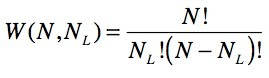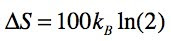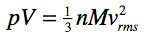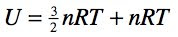## Wednesday, March 31, 2010

### Canonical and localized molecular orbitals

Figure 4.10. 0.045 au isosurfaces of the four valence canonical MOs of NH3 computed using B3LYP/6-31G(d). A value of 0.045 au is chosen because it results in a 0.002 au isodensity surface when squared.
From Molecular Modeling Basics CRC Press, May 2010.
Click on the picture for an interactive version

According to Valence Shell Electron Pair Repulsion (VSEPR) theory many bond angles involving elements such as C, N, and O are close to 109.5o because the four valence electron pairs that surround these atoms adopt a tetrahedral geometry to minimize repulsion. In the case of CH4 the H-C-H angle is exactly 109.5o because the repulsion between the four electron pairs in the C-H bonds are identical. NH3 has a lone pair that is fatter than a bond near the nucleus, so the lone pair-bond repulsion is slightly larger than the bond-bond repulsion. This results in a H-N-H angle of 107o, slightly smaller than 109.5o.

However, this is far from obvious when looking at the four valence MOs of NH3 (Figure 4.10) computed using B3LYP/6-31G(d). The reason is that that MOs are not unique, and that these MOs (which lead to a diagonal Fock matrix and are known as canonical MOs) are not the MOs where the electron pair repulsion is a minimum. Algorithms have been implemented that find a new set of MOs (localized MOs or LMOs) that represent a linear combination of canonical MOs for which the MO–MO repulsion is a minimum (Figure 4.11).

Figure 4.11. 0.045 au isosurfaces of the four valence localized MOs of NH3 computed using B3LYP/6-31G(d). There are three N–H bond LMOs [(a)–(c)] and one lone pair LMO [(d)].
From Molecular Modeling Basics CRC Press, May 2010.
Click on the picture for an interactive version

LMOs for which the inter-orbital repulsion is a minimum are called energy localized orbitals or Edmiston-Ruedenberg orbitals. Other popular choices are Foster-Boys and Pipek-Mezey LMOs, which use different localization criteria.

Using MacMolPlt
The screencast below shows how to compute Ruedenberg LMOs for ammonia using GAMESS (local=ruednbrg in the \$contrl group), and how to display the LMOs, as well as the canonical MOs, in MacMolPlt. I also show how to identify the HOMO and LUMO canonical MOs in MacMolPlt.

Note that I specify a geometry optimization in the GAMESS input file. GAMESS will only compute the LMOs for the optimized geometry.

Using Jmol
I use Jmol for the interactive figures and the scripts can be found here and here. Unlike the density and electrostatic potential, Jmol can generate its own grid data, so to display MO number 2 you simply use "mo 2" in the script. I use
`mo 2; mo cutoff 0.045; mo fill nomesh; mo translucent 0.2`
to make it a little prettier.

Jmol cannot find the MOs in a GAMESS geometry optimization file, and Jmol only stores the LMOs if present. So to display the canonical MOs with Jmol you need a single point energy calculation (output file) and to display the LMOs with Jmol you need a single point energy calculation with local=ruednberg added (output file).

## Wednesday, March 17, 2010

### Shameless book promotion

Things are happening on the book front. A snappy cover (at the top of the blog) and now a promotional flyer - with table of content - which can be downloaded here. The book should be out May 10, complete with non-interactive black-and-white versions of many of the figures found in this blog.## Sunday, March 14, 2010

### Illustrating Entropy

This screencast shows some Molecular Workbench models I made to illustrate entropy.

In the first model a monatomic gas consisting of 100 particles are initially restricted to the left container, but when the separator is removed the gas expands to fill both containers evenly, on average.

The temperature is held constant during the simulation, so the internal energy (which equal to the temperature times a constant) stays constant. The driving force behind the expansion is therefore purely entropic.

Using two simpler cases with two and three particles, I show that the probability of having NL particles (out of N total) on the left iswhere W is the weight or the number of equivalent combinations of particles in two containers. The screencast goes on to show that W is largest when the gas is evenly distributed between the two containers, so that is what happens when the separator is removed because that is the most probable thing to happen.

For N particles and two containers the number of combinations if there are NL particles in the left container is(as explained here and here).

As in the simpler cases, the most likely state for 100 particles is an equal distribution of particles among the two containers (NL = 50). The main difference between 3 and 100 particles is that in the former case you'll occasionally (2/8 or 25 % of the time) see all particles in one container, whereas for 100 particles you'll never (2 x 10-28 % of the time) see all particles in one container.

Entropy, S, is defined aswhere kB is Boltzmann's constant. This function is largest when W is largest, so one can say that the gas expands to maximize its entropy, but it's a bit like saying the gas expands because that is the most probable.

The change in entropy iswhich can be approximated using the more usual equation for the entropy change upon doubling the volume of an ideal gas while keeping the temperature constant(The latter equation becomes more accurate as the number of particles increase, because it relies on Stirling's approximation).

The model is too wide to fit in this blog but you can play around with it on this web page, or you can download the model if you have Molecular Workbench installed on your computer. Enjoy!

Related blog posts
Where does the ln come from in S = k ln(W)?
Entropy, volume, and temperature

## Wednesday, March 3, 2010

### Internal energy and molecular motion

This screencast shows a Molecular Workbench model I made to illustrate the connection between internal energy and molecular motion (the model is included at the bottom of the post).

For a monatomic gas such as He or Ar the part of the internal energy (U) that depends on temperature (T) isand has only contributions from translational motion. Kinetic theory tells us thatwhich, with a little algebra, gives us a direct connection between the internal energy and the average kinetic energy of the atoms in the gas:You can see in the first container that while the internal energy reflects an average speed, the individual atoms can have very different speeds, which are constantly changing due to collisions. This can be illustrated by coloring the atoms according to the kinetic energies (red means more kinetic energy).

Increasing the temperature increases the internal energy and this is reflected in an increase in the average speed, as you can see by clicking on the "Increase T" button.

However, it is important to remember that the internal energy is a reflection of the kinetic energy, which is also a reflection of the mass. Thus, a heavier atoms will have the same internal energy and kinetic energy at the same temperature, but the atoms will move slower on average (the "Increase M" button).

Also, the internal energy can be increase by adding more particles, but this does not change the average speed of the molecules at the same temperature (the "Increase n" button).

The last ("Diatomic") button changes the atoms to diatomic molecules without changing the mass, i.e. the mass of the atoms in the second container are half that of the atoms in the first container. Because the mass is unchanged the average speed of the molecules (more precisely, of their center of mass) is the same as for the atomic gas at the same temperature, as is the translational internal energy (3/2nRT ).

However, notice that the molecules are also rotating, meaning they have additional (internal) energy compared to the atomic gas, which amounts to nRT (3/2nRT for non linear molecules):The internal energy also has a vibrational contribution (here the frequency is converted to a wavenumber, i.e. units of cm-1):though it is hard to make out the vibrational motion in the simulation. However, for most diatomic molecules this contribution is negligible at temperatures relevant to most chemists.

You can play around with the model yourself by clicking on the picture below, or on this web page, or you can download the model if you have Molecular Workbench installed on your computer. Enjoy!